Sie sind hier: ICP » R. Hilfer » Publikationen

VII Inverse Problem

[74.1.1.1] Up to now the direct problem was discussed which consists in finding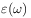for given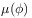and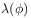from the nonlinear eq. (3.2). [74.1.1.2] The inverse problem is to determine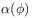orfrom a knowledge of. [74.1.1.3] This is most important for applications such as well logging. [74.1.1.4] Particularly important is the problem of determiningfromandin view of the fact that the local porosity distributioncan be observed much more easily than the local percolation probabilities.

[74.1.2.1] Consider therefore briefly the problem of determiningfrom eq. (3.2) givenand. [74.1.2.2] A general theoretical discussion can be given based on the observation that eq. (3.2) is now linear. [74.1.2.3] It can be written as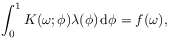(7.1)

where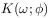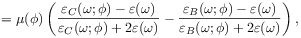(7.2)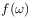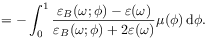(7.3)

[74.1.2.4] Equation (7.1) is a linear Fredholm integral equation of the first kind. [74.1.2.5] Because the kernel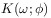is not symmetric, define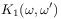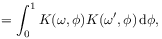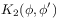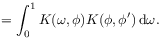[74.1.2.6] General results can be employed to solve eq. (7.1) if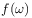is continuous and such that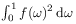exists, and if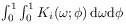exists and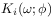is piecewise continuous in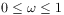,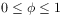. [74.1.2.7] The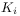are symmetric and have eigenvalues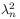. [74.1.2.8] The normal modes called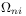and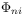can be chosen orthonormal and satisfy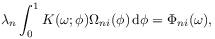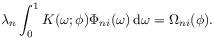[74.2.0.1] These modes are used to solve eq. (7.1). [74.2.0.2] If (7.1) has any solution, then the inhomogeneitycan be written as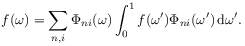(7.4)

[74.2.0.3] It is assumed that the two setsandhave been made orthonormal. [74.2.0.4] Then the solution to eq. (7.1) is given as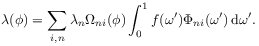(7.5)

[74.2.0.5] The eigenvaluesare the solutions of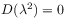, where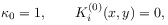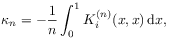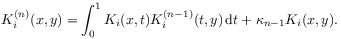[74.2.0.6] These brief remarks about the inverse problem are intended to outline the general characteristics of the problem. [74.2.0.7] A more detailed discussion must await the availability of experimentally observed local porosity distibutions.